# Basic Econometrics Chapter 5 TWOVARIABLE REGRESSION Interval Estimation

• Slides: 32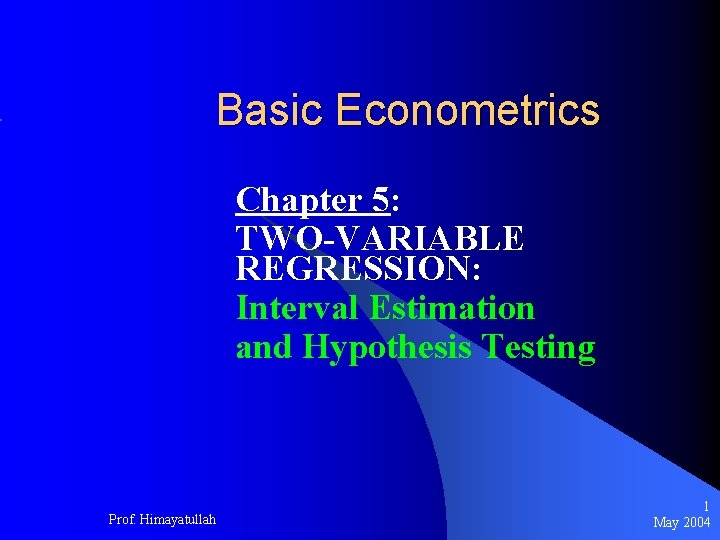Basic Econometrics Chapter 5: TWO-VARIABLE REGRESSION: Interval Estimation and Hypothesis Testing Prof. Himayatullah 1 May 2004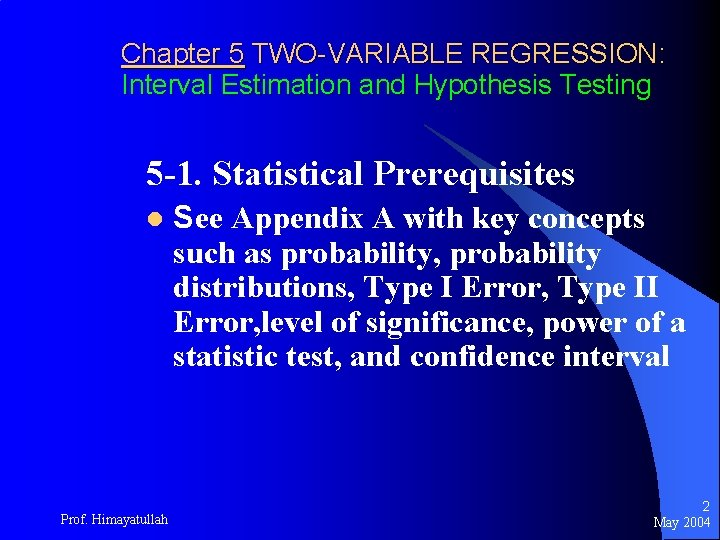Chapter 5 TWO-VARIABLE REGRESSION: Interval Estimation and Hypothesis Testing 5 -1. Statistical Prerequisites l Prof. Himayatullah See Appendix A with key concepts such as probability, probability distributions, Type I Error, Type II Error, level of significance, power of a statistic test, and confidence interval 2 May 2004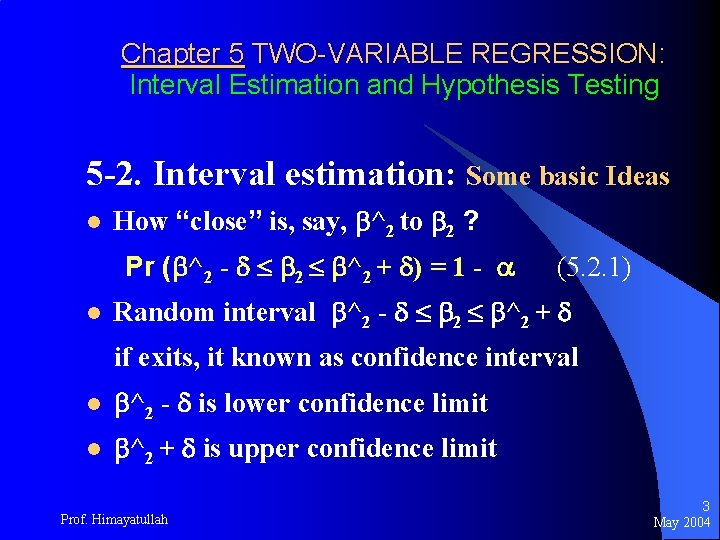Chapter 5 TWO-VARIABLE REGRESSION: Interval Estimation and Hypothesis Testing 5 -2. Interval estimation: Some basic Ideas l How “close” is, say, ^2 to 2 ? Pr ( ^2 - 2 ^2 + ) = 1 - l (5. 2. 1) Random interval ^2 - 2 ^2 + if exits, it known as confidence interval l ^2 - is lower confidence limit l ^2 + is upper confidence limit Prof. Himayatullah 3 May 2004Chapter 5 TWO-VARIABLE REGRESSION: Interval Estimation and Hypothesis Testing 5 -2. Interval estimation: Some basic Ideas l (1 - ) is confidence coefficient, l 0 < < 1 is significance level l Equation (5. 2. 1) does not mean that the Pr of 2 lying between the given limits is (1 - ), but the Pr of constructing an interval that contains 2 is (1 - ) l ( ^2 - , ^2 + ) is random interval Prof. Himayatullah 4 May 2004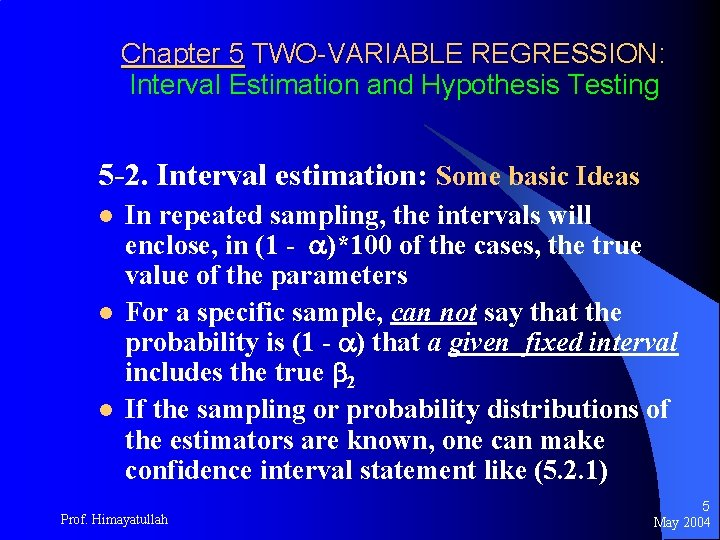Chapter 5 TWO-VARIABLE REGRESSION: Interval Estimation and Hypothesis Testing 5 -2. Interval estimation: Some basic Ideas l l l In repeated sampling, the intervals will enclose, in (1 - )*100 of the cases, the true value of the parameters For a specific sample, can not say that the probability is (1 - ) that a given fixed interval includes the true 2 If the sampling or probability distributions of the estimators are known, one can make confidence interval statement like (5. 2. 1) Prof. Himayatullah 5 May 2004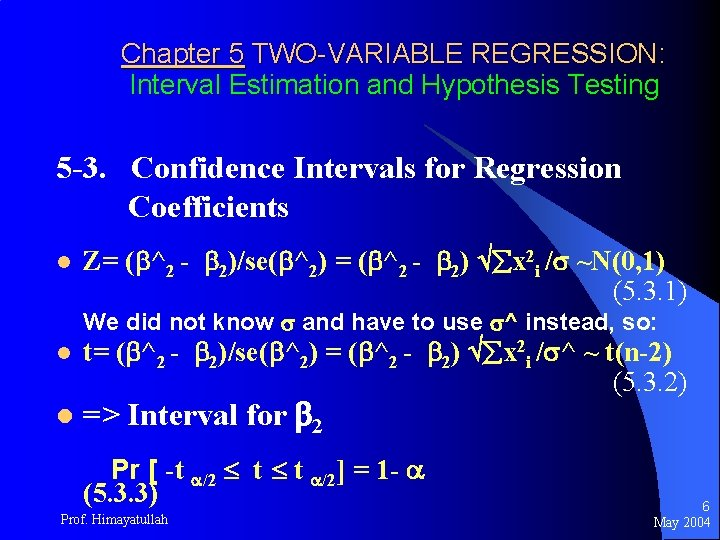Chapter 5 TWO-VARIABLE REGRESSION: Interval Estimation and Hypothesis Testing 5 -3. Confidence Intervals for Regression Coefficients l Z= ( ^2 - 2)/se( ^2) = ( ^2 - 2) x 2 i / ~N(0, 1) (5. 3. 1) We did not know and have to use ^ instead, so: l l t= ( ^2 - 2)/se( ^2) = ( ^2 - 2) x 2 i / ^ ~ t(n-2) (5. 3. 2) => Interval for 2 Pr [ -t /2 t t /2] = 1 - (5. 3. 3) Prof. Himayatullah 6 May 2004Chapter 5 TWO-VARIABLE REGRESSION: Interval Estimation and Hypothesis Testing 5 -3. Confidence Intervals for Regression Coefficients l Or confidence interval for 2 is Pr [ ^2 -t /2 se( ^2) 2 ^2+t /2 se( ^2)] = 1 - (5. 3. 5) l Confidence Interval for 1 Pr [ ^1 -t /2 se( ^1) 1 ^1+t /2 se( ^1)] = 1 - (5. 3. 7) Prof. Himayatullah 7 May 2004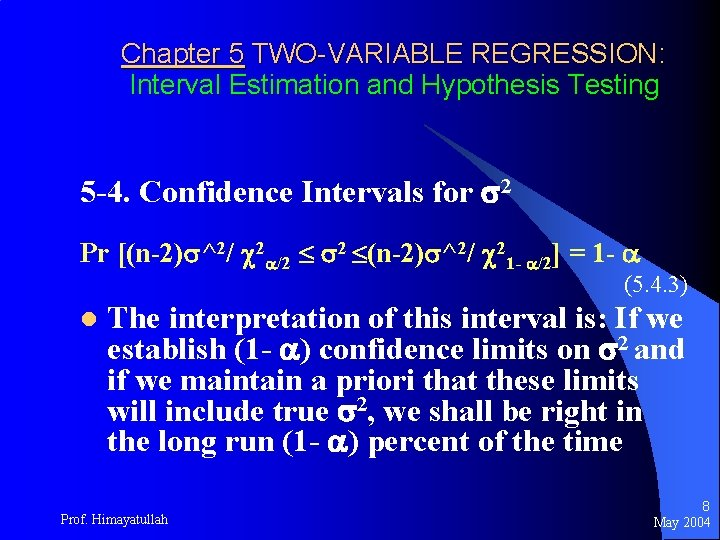Chapter 5 TWO-VARIABLE REGRESSION: Interval Estimation and Hypothesis Testing 5 -4. Confidence Intervals for 2 Pr [(n-2) ^2/ 2 /2 2 (n-2) ^2/ 21 - /2] = 1 - (5. 4. 3) l The interpretation of this interval is: If we establish (1 - ) confidence limits on 2 and if we maintain a priori that these limits will include true 2, we shall be right in the long run (1 - ) percent of the time Prof. Himayatullah 8 May 2004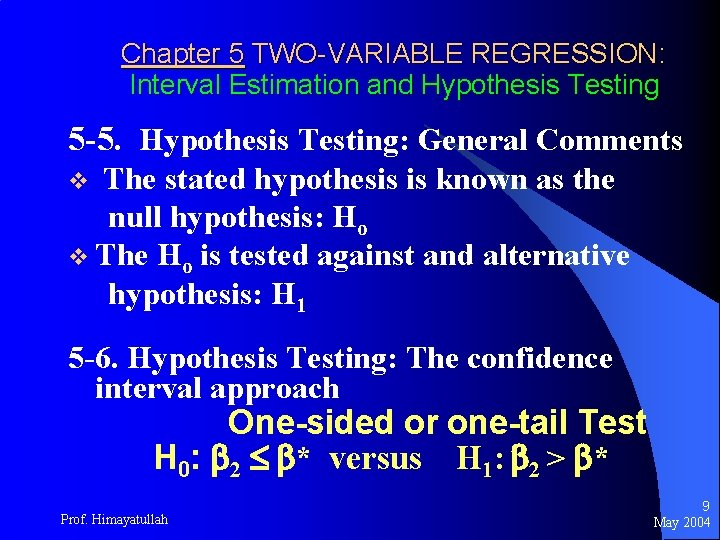Chapter 5 TWO-VARIABLE REGRESSION: Interval Estimation and Hypothesis Testing 5 -5. Hypothesis Testing: General Comments The stated hypothesis is known as the null hypothesis: Ho v The Ho is tested against and alternative hypothesis: H 1 v 5 -6. Hypothesis Testing: The confidence interval approach One-sided or one-tail Test H 0: 2 * versus H 1: 2 > * Prof. Himayatullah 9 May 2004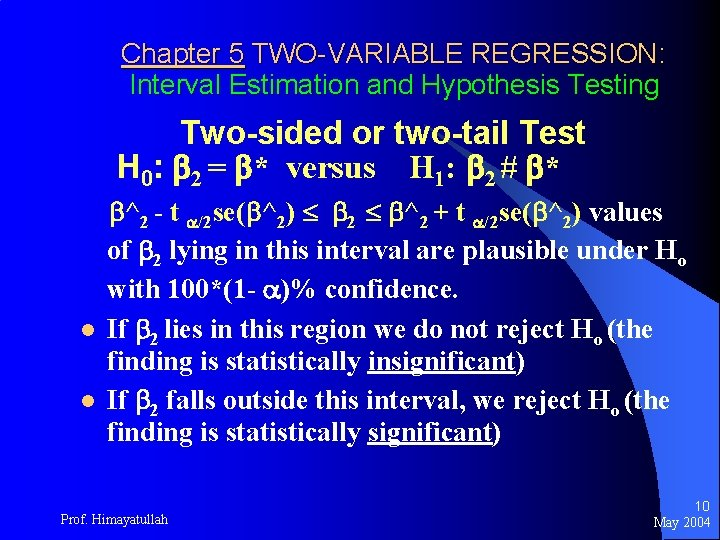Chapter 5 TWO-VARIABLE REGRESSION: Interval Estimation and Hypothesis Testing Two-sided or two-tail Test H 0: 2 = * versus H 1: 2 # * l l ^2 - t /2 se( ^2) 2 ^2 + t /2 se( ^2) values of 2 lying in this interval are plausible under Ho with 100*(1 - )% confidence. If 2 lies in this region we do not reject Ho (the finding is statistically insignificant) If 2 falls outside this interval, we reject Ho (the finding is statistically significant) Prof. Himayatullah 10 May 2004Chapter 5 TWO-VARIABLE REGRESSION: Interval Estimation and Hypothesis Testing 5 -7. Hypothesis Testing: The test of significance approach A test of significance is a procedure by which sample results are used to verify the truth or falsity of a null hypothesis l Testing the significance of regression coefficient: The t-test Pr [ ^2 -t /2 se( ^2) 2 ^2+t /2 se( ^2)]= 1 (5. 7. 2) Prof. Himayatullah 11 May 2004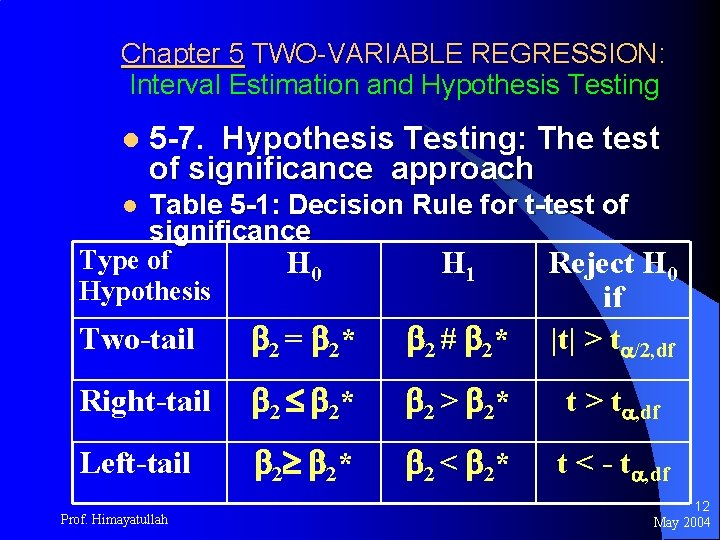Chapter 5 TWO-VARIABLE REGRESSION: Interval Estimation and Hypothesis Testing l 5 -7. Hypothesis Testing: The test of significance approach Table 5 -1: Decision Rule for t-test of significance Type of H 0 H 1 Reject H 0 Hypothesis if l Two-tail 2 = 2* 2 # 2* |t| > t /2, df Right-tail 2 2* 2 > 2* t > t , df Left-tail 2 2* 2 < 2* t < - t , df Prof. Himayatullah 12 May 2004Chapter 5 TWO-VARIABLE REGRESSION: Interval Estimation and Hypothesis Testing l 5 -7. Hypothesis Testing: The test of significance approach Testing the significance of 2 : The 2 Test Under the Normality assumption we have: 2 = ^2 (n-2) ------- ~ 2 (n-2) 2 (5. 4. 1) From (5. 4. 2) and (5. 4. 3) on page 520 => Prof. Himayatullah 13 May 2004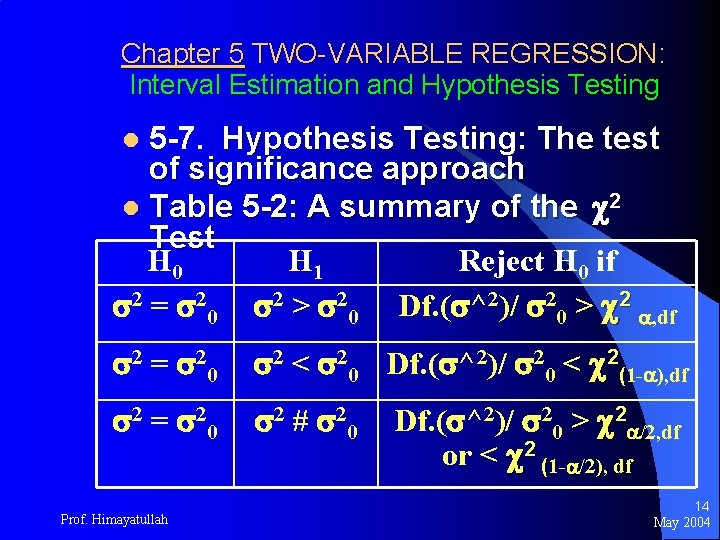Chapter 5 TWO-VARIABLE REGRESSION: Interval Estimation and Hypothesis Testing 5 -7. Hypothesis Testing: The test of significance approach l Table 5 -2: A summary of the 2 Test H 0 H 1 Reject H 0 if 2 = 20 2 > 20 Df. ( ^2)/ 20 > 2 , df l 2 = 20 2 < 20 Df. ( ^2)/ 20 < 2(1 - ), df 2 = 20 2 # 20 Prof. Himayatullah Df. ( ^2)/ 20 > 2 /2, df or < 2 (1 - /2), df 14 May 2004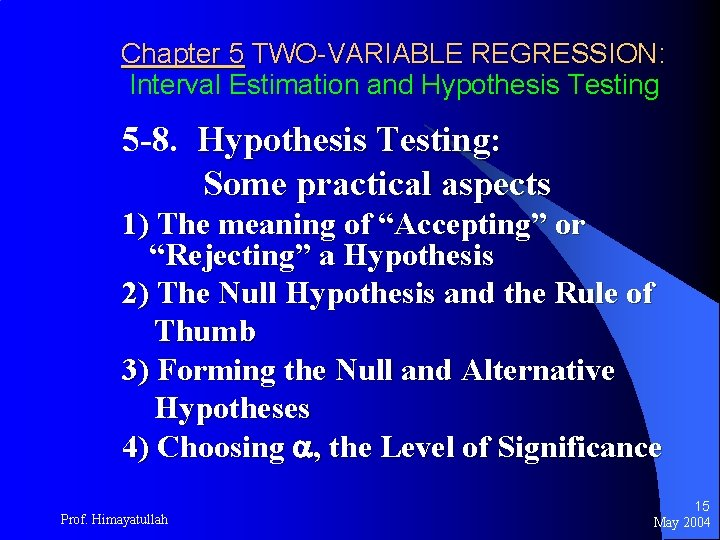Chapter 5 TWO-VARIABLE REGRESSION: Interval Estimation and Hypothesis Testing 5 -8. Hypothesis Testing: Some practical aspects 1) The meaning of “Accepting” or “Rejecting” a Hypothesis 2) The Null Hypothesis and the Rule of Thumb 3) Forming the Null and Alternative Hypotheses 4) Choosing , the Level of Significance Prof. Himayatullah 15 May 2004Chapter 5 TWO-VARIABLE REGRESSION: Interval Estimation and Hypothesis Testing 5 -8. Hypothesis Testing: Some practical aspects 5) The Exact Level of Significance: The p-Value [See page 132] 6) Statistical Significance versus Practical Significance 7) The Choice between Confidence. Interval and Test-of-Significance Approaches to Hypothesis Testing [Warning: Read carefully pages 117 -134 ] Prof. Himayatullah 16 May 2004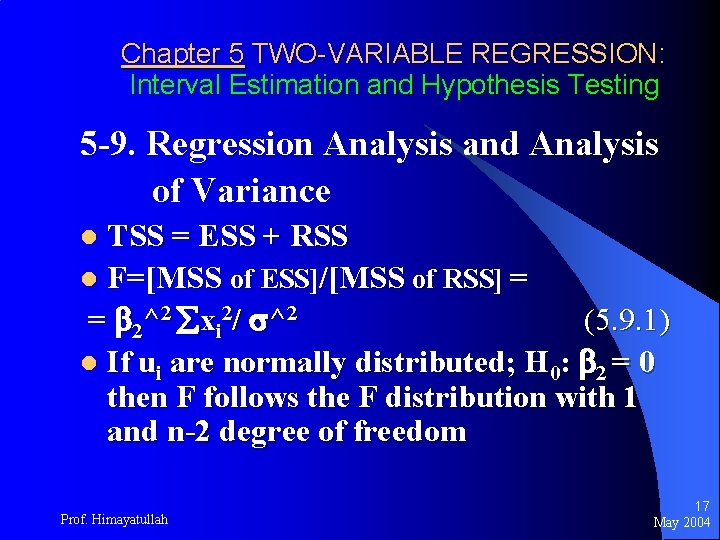Chapter 5 TWO-VARIABLE REGRESSION: Interval Estimation and Hypothesis Testing 5 -9. Regression Analysis and Analysis of Variance TSS = ESS + RSS l F=[MSS of ESS]/[MSS of RSS] = = 2^2 xi 2/ ^2 (5. 9. 1) l If ui are normally distributed; H 0: 2 = 0 then F follows the F distribution with 1 and n-2 degree of freedom l Prof. Himayatullah 17 May 2004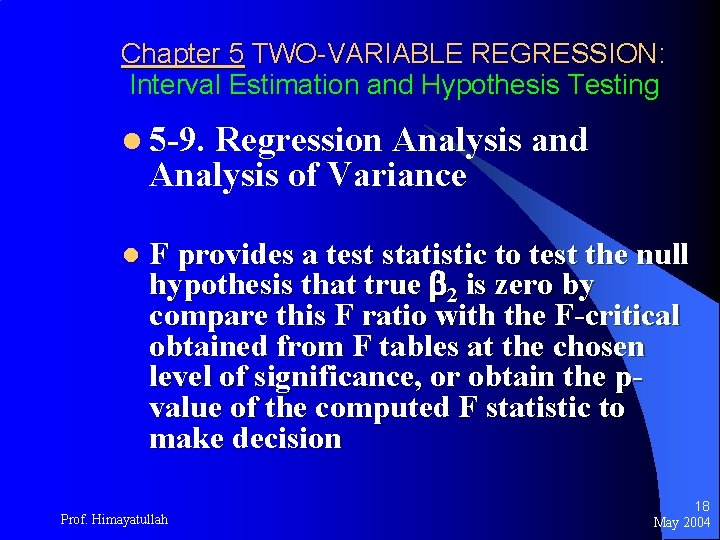Chapter 5 TWO-VARIABLE REGRESSION: Interval Estimation and Hypothesis Testing l 5 -9. Regression Analysis and Analysis of Variance l F provides a test statistic to test the null hypothesis that true 2 is zero by compare this F ratio with the F-critical obtained from F tables at the chosen level of significance, or obtain the pvalue of the computed F statistic to make decision Prof. Himayatullah 18 May 2004Chapter 5 TWO-VARIABLE REGRESSION: Interval Estimation and Hypothesis Testing l 5 -9. Regression Analysis and Analysis of Variance l Table 5 -3. ANOVA for two-variable regression model Source of Variation Sum of square ( SS) Degree of Freedom (Df) ESS (due to regression) y^i 2 = 2^2 xi 2 1 RSS (due to residuals) u^i 2 n-2 TSS y i 2 n-1 Prof. Himayatullah Mean sum of square ( MSS) 2^2 xi 2 u^i 2 /(n-2)= ^2 19 May 2004Chapter 5 TWO-VARIABLE REGRESSION: Interval Estimation and Hypothesis Testing 5 -10. Application of Regression Analysis: Problem of Prediction l l By the data of Table 3 -2, we obtained the sample regression (3. 6. 2) : Y^i = 24. 4545 + 0. 5091 Xi , where Y^i is the estimator of true E(Yi) There are two kinds of prediction as follows: Prof. Himayatullah 20 May 2004Chapter 5 TWO-VARIABLE REGRESSION: Interval Estimation and Hypothesis Testing 5 -10. Application of Regression Analysis: Problem of Prediction l l Mean prediction: Prediction of the conditional mean value of Y corresponding to a chosen X, say X 0, that is the point on the population regression line itself (see pages 137 -138 for details) Individual prediction: Prediction of an individual Y value corresponding to X 0 (see pages 138 -139 for details) Prof. Himayatullah 21 May 2004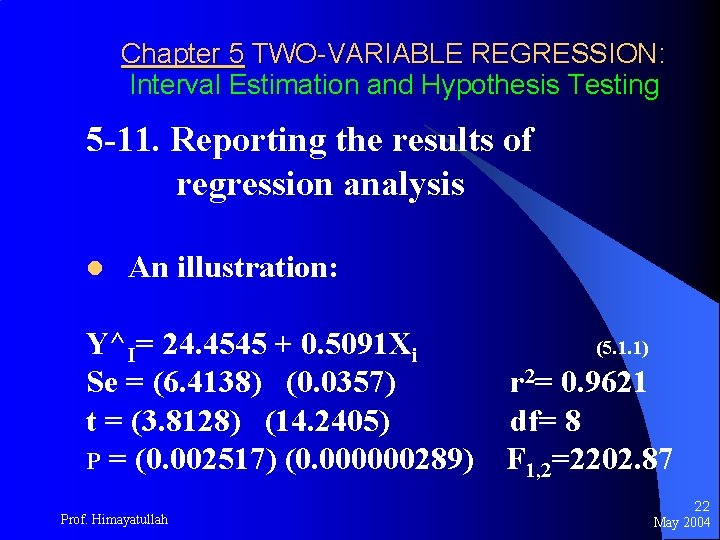Chapter 5 TWO-VARIABLE REGRESSION: Interval Estimation and Hypothesis Testing 5 -11. Reporting the results of regression analysis l An illustration: Y^I= 24. 4545 + 0. 5091 Xi Se = (6. 4138) (0. 0357) t = (3. 8128) (14. 2405) P = (0. 002517) (0. 000000289) Prof. Himayatullah (5. 1. 1) r 2= 0. 9621 df= 8 F 1, 2=2202. 87 22 May 2004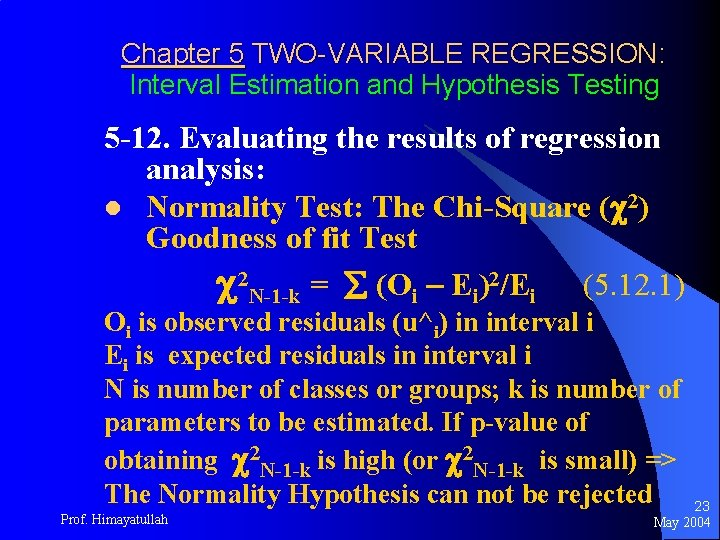Chapter 5 TWO-VARIABLE REGRESSION: Interval Estimation and Hypothesis Testing 5 -12. Evaluating the results of regression analysis: l Normality Test: The Chi-Square ( 2) Goodness of fit Test 2 N-1 -k = (Oi – Ei)2/Ei (5. 12. 1) Oi is observed residuals (u^i) in interval i Ei is expected residuals in interval i N is number of classes or groups; k is number of parameters to be estimated. If p-value of obtaining 2 N-1 -k is high (or 2 N-1 -k is small) => The Normality Hypothesis can not be rejected Prof. Himayatullah 23 May 2004Chapter 5 TWO-VARIABLE REGRESSION: Interval Estimation and Hypothesis Testing 5 -12. Evaluating the results of regression analysis: l Normality Test: The Chi-Square ( 2) Goodness of fit Test H 0: ui is normally distributed H 1: ui is un-normally distributed Calculated- 2 N-1 -k = (Oi – Ei)2/Ei (5. 12. 1) Decision rule: Calculated- 2 N-1 -k > Critical- 2 N-1 -k then H 0 can be rejected Prof. Himayatullah 24 May 2004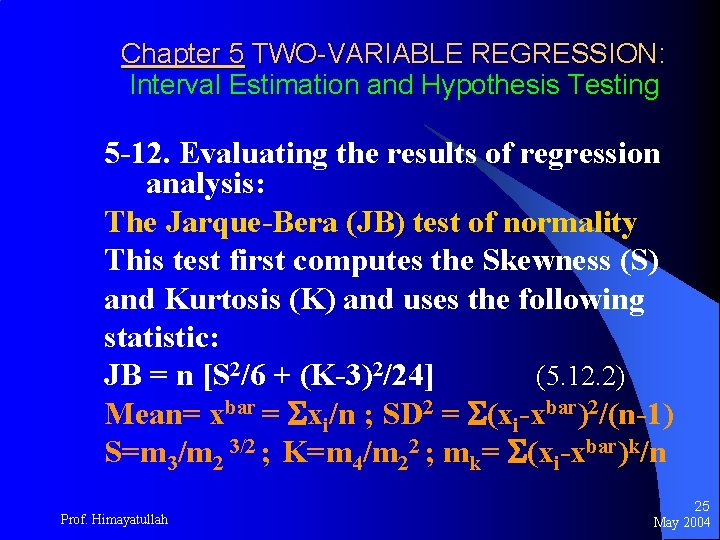Chapter 5 TWO-VARIABLE REGRESSION: Interval Estimation and Hypothesis Testing 5 -12. Evaluating the results of regression analysis: The Jarque-Bera (JB) test of normality This test first computes the Skewness (S) and Kurtosis (K) and uses the following statistic: JB = n [S 2/6 + (K-3)2/24] (5. 12. 2) Mean= xbar = xi/n ; SD 2 = (xi-xbar)2/(n-1) S=m 3/m 2 3/2 ; K=m 4/m 22 ; mk= (xi-xbar)k/n Prof. Himayatullah 25 May 2004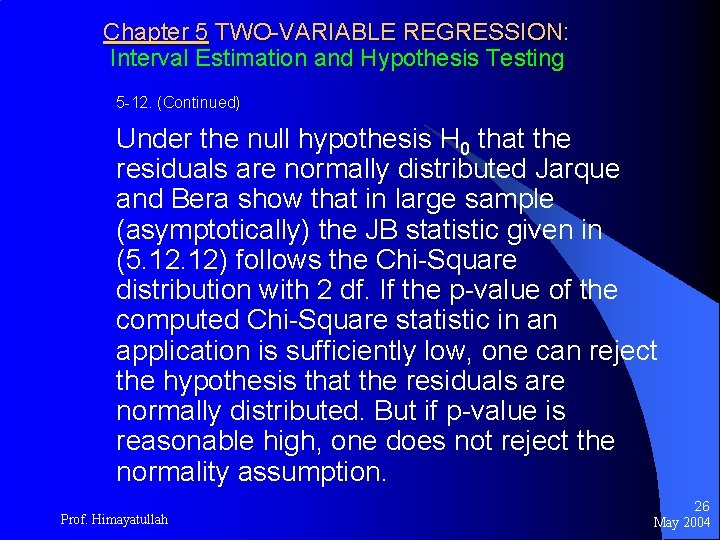Chapter 5 TWO-VARIABLE REGRESSION: Interval Estimation and Hypothesis Testing 5 -12. (Continued) Under the null hypothesis H 0 that the residuals are normally distributed Jarque and Bera show that in large sample (asymptotically) the JB statistic given in (5. 12) follows the Chi-Square distribution with 2 df. If the p-value of the computed Chi-Square statistic in an application is sufficiently low, one can reject the hypothesis that the residuals are normally distributed. But if p-value is reasonable high, one does not reject the normality assumption. Prof. Himayatullah 26 May 2004Chapter 5 TWO-VARIABLE REGRESSION: Interval Estimation and Hypothesis Testing 5 -13. Summary and Conclusions 1. Estimation and Hypothesis testing constitute the two main branches of classical statistics 2. Hypothesis testing answers this question: Is a given finding compatible with a stated hypothesis or not? 3. There are two mutually complementary approaches to answering the preceding question: Confidence interval and test of significance. Prof. Himayatullah 27 May 2004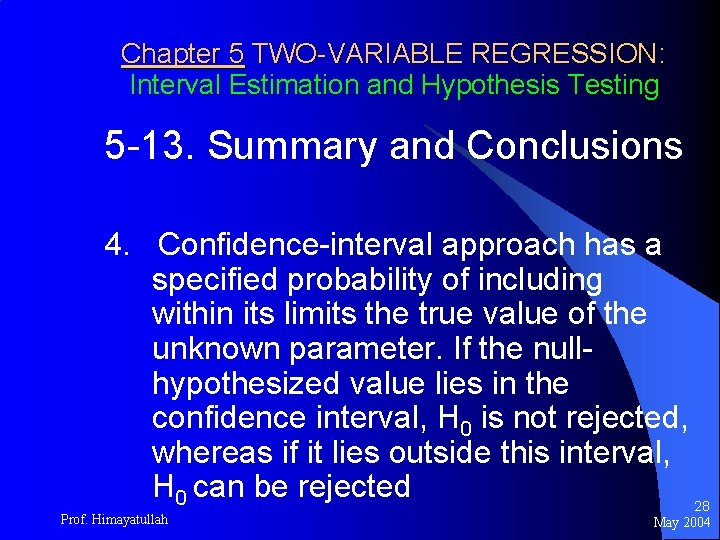Chapter 5 TWO-VARIABLE REGRESSION: Interval Estimation and Hypothesis Testing 5 -13. Summary and Conclusions 4. Confidence-interval approach has a specified probability of including within its limits the true value of the unknown parameter. If the nullhypothesized value lies in the confidence interval, H 0 is not rejected, whereas if it lies outside this interval, H 0 can be rejected 28 Prof. Himayatullah May 2004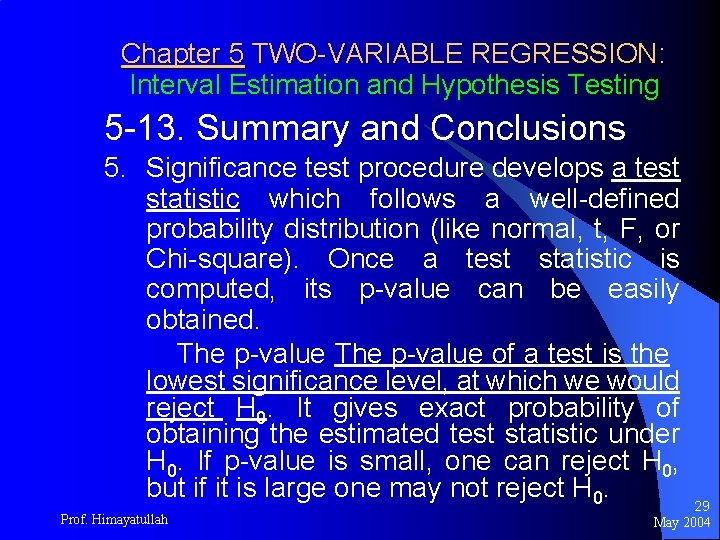Chapter 5 TWO-VARIABLE REGRESSION: Interval Estimation and Hypothesis Testing 5 -13. Summary and Conclusions 5. Significance test procedure develops a test statistic which follows a well-defined probability distribution (like normal, t, F, or Chi-square). Once a test statistic is computed, its p-value can be easily obtained. The p-value of a test is the lowest significance level, at which we would reject H 0. It gives exact probability of obtaining the estimated test statistic under H 0. If p-value is small, one can reject H 0, but if it is large one may not reject H 0. Prof. Himayatullah 29 May 2004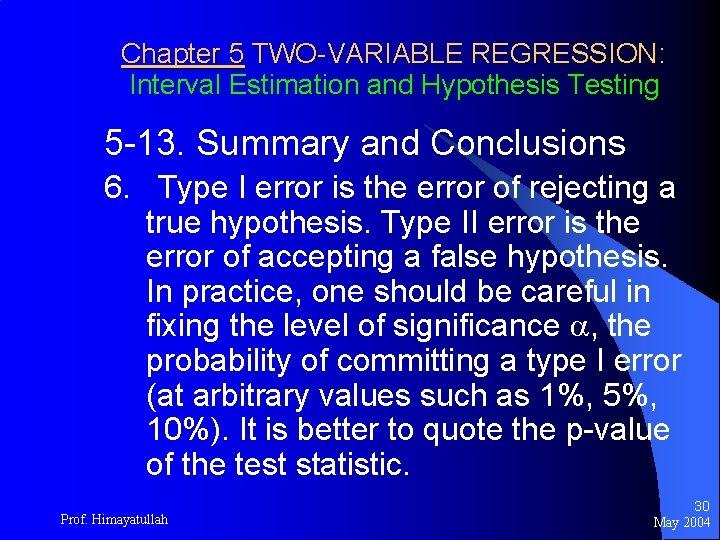Chapter 5 TWO-VARIABLE REGRESSION: Interval Estimation and Hypothesis Testing 5 -13. Summary and Conclusions 6. Type I error is the error of rejecting a true hypothesis. Type II error is the error of accepting a false hypothesis. In practice, one should be careful in fixing the level of significance , the probability of committing a type I error (at arbitrary values such as 1%, 5%, 10%). It is better to quote the p-value of the test statistic. Prof. Himayatullah 30 May 2004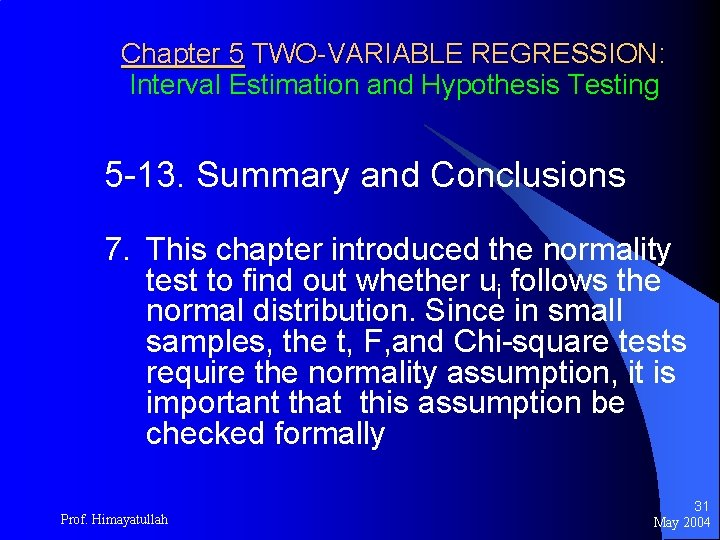Chapter 5 TWO-VARIABLE REGRESSION: Interval Estimation and Hypothesis Testing 5 -13. Summary and Conclusions 7. This chapter introduced the normality test to find out whether ui follows the normal distribution. Since in small samples, the t, F, and Chi-square tests require the normality assumption, it is important that this assumption be checked formally Prof. Himayatullah 31 May 2004Chapter 5 TWO-VARIABLE REGRESSION: Interval Estimation and Hypothesis Testing 5 -13. Summary and Conclusions (ended) 8. If the model is deemed practically adequate, it may be used forecasting purposes. But should not go too far out of the sample range of the regressor values. Otherwise, forecasting errors can increase dramatically. Prof. Himayatullah 32 May 2004